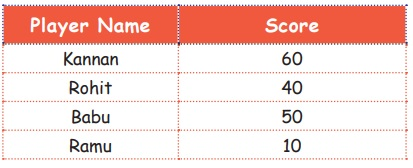Home | | Maths 4th Std | Exercise 6.2 (Collect and represent data in the form of bar graphs)

# Exercise 6.2 (Collect and represent data in the form of bar graphs)

Text Book Back Exercises Questions with Answers, Solution : 4th Maths : Term 1 Unit 6 : Information Processing : Exercise 6.2 (Collect and represent data in the form of bar graphs)

Exercise 6.2

1. Bar diagram of a student’s first term scores are given.a. Which subject is the highest score in “A” ?

Maths

b. Which subject is the lowest score in “A” ?

English

c. Which subject is the same score in “A” ?

Tamil and Science

2. The score card of the players in a cricket match is given.Draw a Bar diagram.Tags : Information Processing | Term 1 Chapter 6 | 4th Maths , 4th Maths : Term 1 Unit 6 : Information Processing
Study Material, Lecturing Notes, Assignment, Reference, Wiki description explanation, brief detail
4th Maths : Term 1 Unit 6 : Information Processing : Exercise 6.2 (Collect and represent data in the form of bar graphs) | Information Processing | Term 1 Chapter 6 | 4th Maths